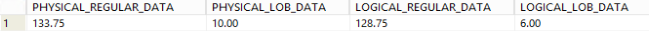## Find database size allocated for LOB data in Db2 database

Article for:

Query below can help you find how much space is allocated for LOB data.

## Query

``````select dec(sum(data_object_p_size
+ long_object_p_size
+ xml_object_p_size)/1024.0,20,2) as physical_regular_data,
dec(sum(lob_object_p_size)/1024, 20,2) as physical_lob_data,
dec(sum(data_object_l_size
+ long_object_l_size
+ xml_object_l_size)/1024.0,20,2) as logical_regular_data,
dec(sum(lob_object_l_size/1024), 20,2) as logical_lob_data
where tabschema not like 'SYS%'
``````

## Columns

• physical_regular_data - amount of disk space that is physically allocated for the regular data
• physical_lob_data - amount of disk space that is physically allocated for the LOB data
• logical_regular_data - amount of disk space logically allocated for the regular data
• logical_lob_data - amount of disk space logically allocated for the LOB data

## Rows

• Query returns just one row

## Sample results

Space allocation for LOB and other data types: